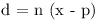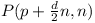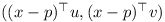# Rigid body game physics 5

In order to handle collisions and resting contacts, it is necessary to determine contact points. One can achieve this using the separating plane algorithm (also see Baraff).

## Separating Planes Algorithm

The separating plane algorithm only applies to convex polyhedra. However non-convex objects can in many cases be approximated by a union of convex polyhedra. A separating plane is a plane such that all points of the polyhedra A and B lie on opposite sides of the plane. If two polyhedra are not intersecting, a separating plane can either be defined using one of the faces of one of the polyhedra or be constructed from one edge of A and one edge of B.

The distance d of a point x from the plane P(p,n) defined by the point p and the normal vector n is:1. In the case of using a face from polyhedron A, the point p is a corner of the face. The normal vector n is the normalized cross product of two edges of the face and pointing outward from the polyhedron. The point x of polyhedron B with the smallest distance d defines the distance of B to the face of A. If this distance is positive, the face of polyhedron A is a separating plane.

2. In the case of using two edges, the point p is the corner of an edge from polyhedron A. The normal vector n is the positive or negative normalized cross product of the two edge vectors. Again the point x of polyhedron B with the smallest distance d defines the distance of B to the face of A.

From all the planes, the one with the largest distance d is selected. If the distance is zero or negative, the two polyhedra are in contact. One can place a plane in the "middle" of the contact by moving it by half the distance d, i.e.In the next step all points from A and B, which have a distance less than ε to the plane, are selected. Two vectors u and v orthogonal to the normal n and orthogonal to each other are determined. The points are projected onto the plane and represented using the vectors u and v:The convex hull of the each of the two polygons is determined. Then the intersection of the resulting two 2D polygons is computed. Finally the convex hull of the intersection is taken. The points are projected back into 3D space. These are the contact points used for simulating the colliding and resting contacts.